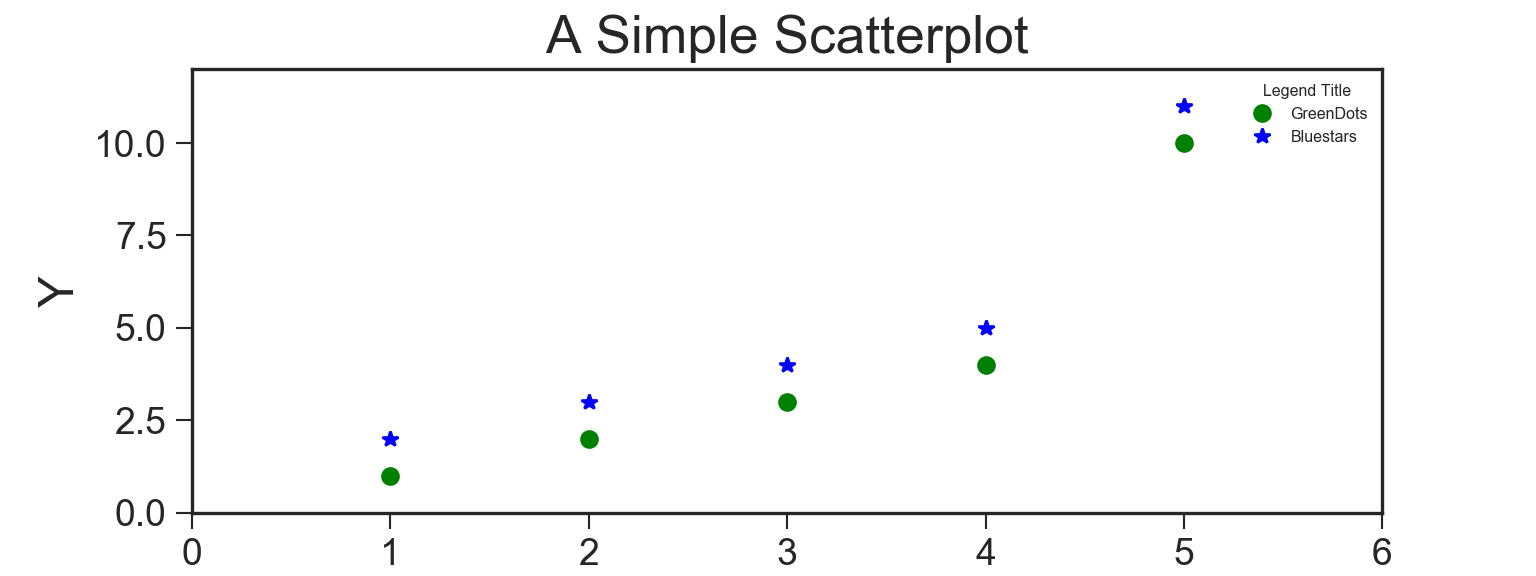## Graphing in Python - a walkthrough

November 07 2019## Plotnine

One of the strengths of the R language is its very powerful graphing package `ggplot2`, with its distinctive implementation of Leland Wilkinson’s Grammar of Graphics. The `Plotnine` package brings this (at least part of it, it seems for now) capability to Python, with very little change to the R syntax.

Here is a (ever growing) collection of useful links curated by us to get your started with using `Plotnine`.

The brackets around the `ggplot()` function looks strange at first, but it is needed for the signature multi-line `ggplot2` grammer to work:

``````import pandas as pd
from plotnine import *
from random import randint

# 100 random numbers
random_numbers = [randint(1, 100) for p in range(0, 100)]

# Create DataFrame
df = pd.DataFrame({'number': random_numbers})

# Draw plot
p = (
ggplot(df, aes(x='number')) +
geom_histogram(bins=20, na_rm=True) +
ggtitle('Histogram of random numbers') +
theme_light()
)

p.draw();``````If you want to save the plot to file:

``p.save("output.png")``

### In Streamlit

And just because I can see myself wanting to use these plots in a Streamlit app:

``````import pandas as pd
import numpy as np
from plotnine import *
import streamlit as st

n = 10

df = pd.DataFrame({'x': np.arange(n),
'y': np.arange(n),
'yfit': np.arange(n) + np.tile([-.2, .2], n // 2),
'cat': ['a', 'b'] * (n // 2)})

a = (ggplot(df)
+ geom_col(aes('x', 'y'))
)

fig = a.draw();   ## Needed to remove the "ggplot<#>" message

st.pyplot()``````